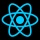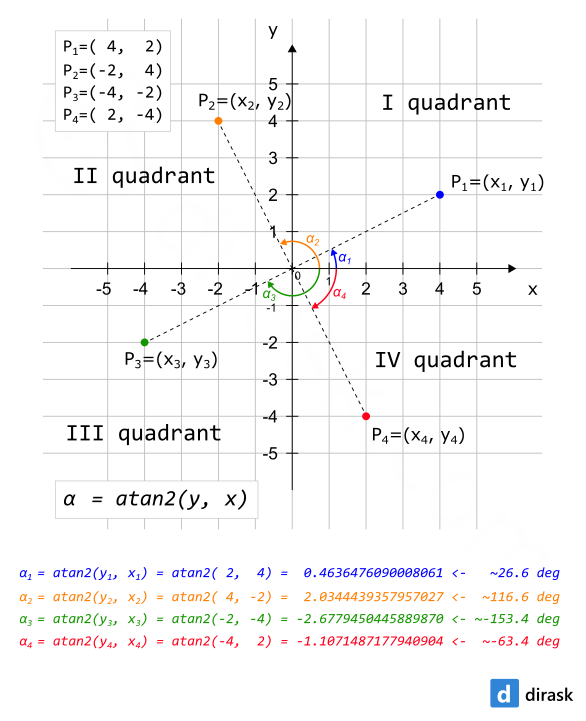Home
IT Knowledge
Inspiration
Languages
EN

# Python - math.atan2() method example

0 points
Created by:Admin Dirask Community
1270

The `math.atan2()` function returns the angle in radians in the range `-math.pi/2` to `+math.pi/2` between the positive x-axis and the ray to the point (x, y) ≠ (0, 0).

``````import math

#                y    x         angle in radians
print(math.atan2(2,   4))     # 0.4636476090008061  <-   ~26.6 degrees
print(math.atan2(4,  -2))     # 2.0344439357957027  <-  ~116.6 degrees
print(math.atan2(-2, -4))     # -2.677945044588987  <- ~-153.4 degrees
print(math.atan2(-4,  2))     # -1.1071487177940904 <-  ~-63.4 degrees``````

`atan2()` method has been visualized on below image:Atan2(y, x) function visualization - Python math module.

## 1. Documentation

 Syntax ``math.atan2(y, x)`` Parameters `y`, `x` - double values that are coordinates of the point. Result `number` value of the angle between two lines `OP` and `OX` in radians in the range `-math.pi/2` to `+math.pi/2`. Where: `O=(0, 0)` - intersection point of coordinate system axes,`P=(x, y)` - out point,`OX` - positive part of x-axis. If point is in the 1st (I) or 2nd (II) quadrant angle is measured in a counterclockwise direction. If point is in the 3rd (III) or 4th (IV) quadrant angle is measured in a clockwise direction. Note: angle arrows show measured angles for points located in different quadrants. Description `atan2` is a method that takes two parameters and returns an approximation of the arctangent(y/x) function taking into account quadrants of a point location (`P=(x, y)`).

## 2. Working with degrees

``````import math

def calculate_angle(x, y):
angle = math.atan2(x, y)
return (180 / math.pi) * angle

#                     y    x       degrees
print(calculate_angle(2,   4))   # 26.56505117707799
print(calculate_angle(4,  -2))   # 116.56505117707799
print(calculate_angle(-2, -4))   # -153.434948822922
print(calculate_angle(-4,  2))   # -63.43494882292201``````

## 3.  Conversion to only clockwise angles in degrees

This section shows how to convert angles to clockwise angles (from 0 to 360 degrees).

``````import math

def calculate_angle(x, y):
angle = math.atan2(x, y)

if angle < 0:
angle += 2 * math.pi
return (180 / math.pi) * angle

#                     y    x        degrees
print(calculate_angle(2,   4))    # 26.56505117707799
print(calculate_angle(4,  -2))    # 116.56505117707799
print(calculate_angle(-2, -4))    # 206.565051177078
print(calculate_angle(-4,  2))    # 296.565051177078
``````

## 4.  Conversion to only counterclockwise angles in degrees

This section shows how to convert angles to counterclockwise angles (from -360 to 0 degrees).

``````import math

def calculate_angle(x, y):
angle = math.atan2(x, y)

if angle > 0:
angle -= 2 * math.pi
return (180 / math.pi) * angle  # rad to deg conversion

#                     y   x        degrees
print(calculate_angle(2,   4))    # -333.434948822922
print(calculate_angle(4,  -2))    # -243.434948822922
print(calculate_angle(-2, -4))    # -153.434948822922
print(calculate_angle(-4,  2))    # -63.43494882292201``````

## Python - math module

##### 🚀
Get your tech brand or product in front of software developers.
For more information Contact us

Join